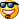# Moving Entire Rows in Excel

I had an interesting request today that I think is worth sharing.I was essentially asked, what the most efficient way of moving a row from one point in a worksheet to a lower point in the sheet. Such as moving Row 6 to Row 42. I developed the following method to do this:

private bool MoveRow(Excel.Range rngRowToMove, int moveRowNum)
{
// get reference to the sheet
Excel.Application xlApp = rngRowToMove.Application;
Excel.Worksheet ws = (Excel.Worksheet)rngRowToMove.Application.ActiveSheet;
int rowToDelete = rngRowToMove.Row; // and the original row number

// verify that the moveRowNum is further down (below/higher number) than
// the rngRowToMove location. If it is not then we fail…
if (moveRowNum <= rowToDelete)
return false;

try
{
// now grab the row where we want to move to
Excel.Range rngMoveTo = ((Excel.Range)rngRowToMove.Application.Cells[moveRowNum, 1]).EntireRow;
// insert a row so as to not delete the data already there
// but only do it if it is not the last row in the sheet
if(moveRowNum < ws.UsedRange.Rows.Count)
rngMoveTo.Insert(Excel.XlInsertShiftDirection.xlShiftDown, Excel.XlInsertFormatOrigin.xlFormatFromLeftOrAbove);
// and move…
rngRowToMove.EntireRow.Cut(rngMoveTo);
// now go to delete the empty row
((Excel.Range)xlApp.Cells[rowToDelete, 1]).EntireRow.Delete(Excel.XlDeleteShiftDirection.xlShiftUp);
return true;
}
catch
{
return false;
}
}

To call this you can use a line like this:

MoveRow(xlApp.ActiveCell, ((Excel.Worksheet)xlApp.ActiveSheet).UsedRange.Rows.Count + 1)

What this does is moves the currently selected row (if the selection is anywhere in a given row), to the very last row of the sheet.